# Inheritance in Java

Inheritance is an important pillar of OOP(Object Oriented Programming). It is the mechanism in java by which one class is allow to inherit the features(fields and methods) of another class.
Important terminology:

• Super Class: The class whose features are inherited is known as super class(or a base class or a parent class).
• Sub Class: The class that inherits the other class is known as sub class(or a derived class, extended class, or child class). The subclass can add its own fields and methods in addition to the superclass fields and methods.
• Reusability: Inheritance supports the concept of “reusability”, i.e. when we want to create a new class and there is already a class that includes some of the code that we want, we can derive our new class from the existing class. By doing this, we are reusing the fields and methods of the existing class.

How to use inheritance in Java

The keyword used for inheritance is extends.
Syntax :

```class derived-class extends base-class
{
//methods and fields
}
```

Example: In below example of inheritance, class Bicycle is a base class, class MountainBike is a derived class which extends Bicycle class and class Test is a driver class to run program.

 `//Java program to illustrate the  ` `// concept of inheritance ` ` `  `// base class ` `class` `Bicycle  ` `{ ` `    ``// the Bicycle class has two fields ` `    ``public` `int` `gear; ` `    ``public` `int` `speed; ` `         `  `    ``// the Bicycle class has one constructor ` `    ``public` `Bicycle(``int` `gear, ``int` `speed) ` `    ``{ ` `        ``this``.gear = gear; ` `        ``this``.speed = speed; ` `    ``} ` `         `  `    ``// the Bicycle class has three methods ` `    ``public` `void` `applyBrake(``int` `decrement) ` `    ``{ ` `        ``speed -= decrement; ` `    ``} ` `         `  `    ``public` `void` `speedUp(``int` `increment) ` `    ``{ ` `        ``speed += increment; ` `    ``} ` `     `  `    ``// toString() method to print info of Bicycle ` `    ``public` `String toString()  ` `    ``{ ` `        ``return``(``"No of gears are "``+gear ` `                ``+``"\n"` `                ``+ ``"speed of bicycle is "``+speed); ` `    ``}  ` `} ` ` `  `// derived class ` `class` `MountainBike ``extends` `Bicycle  ` `{ ` `     `  `    ``// the MountainBike subclass adds one more field ` `    ``public` `int` `seatHeight; ` ` `  `    ``// the MountainBike subclass has one constructor ` `    ``public` `MountainBike(``int` `gear,``int` `speed, ` `                        ``int` `startHeight) ` `    ``{ ` `        ``// invoking base-class(Bicycle) constructor ` `        ``super``(gear, speed); ` `        ``seatHeight = startHeight; ` `    ``}  ` `         `  `    ``// the MountainBike subclass adds one more method ` `    ``public` `void` `setHeight(``int` `newValue) ` `    ``{ ` `        ``seatHeight = newValue; ` `    ``}  ` `     `  `    ``// overriding toString() method ` `    ``// of Bicycle to print more info ` `    ``@Override` `    ``public` `String toString() ` `    ``{ ` `        ``return` `(``super``.toString()+ ` `                ``"\nseat height is "``+seatHeight); ` `    ``} ` `     `  `} ` ` `  `// driver class ` `public` `class` `Test  ` `{ ` `    ``public` `static` `void` `main(String args[])  ` `    ``{ ` `         `  `        ``MountainBike mb = ``new` `MountainBike(``3``, ``100``, ``25``); ` `        ``System.out.println(mb.toString()); ` `             `  `    ``} ` `} `

Output:

```No of gears are 3
speed of bicycle is 100
seat height is 25
```

In above program, when an object of MountainBike class is created, a copy of the all methods and fields of the superclass acquire memory in this object. That is why, by using the object of the subclass we can also access the members of a superclass.
Please note that during inheritance only object of subclass is created, not the superclass. For more, refer Java Object Creation of Inherited Class.
Illustrative image of the program: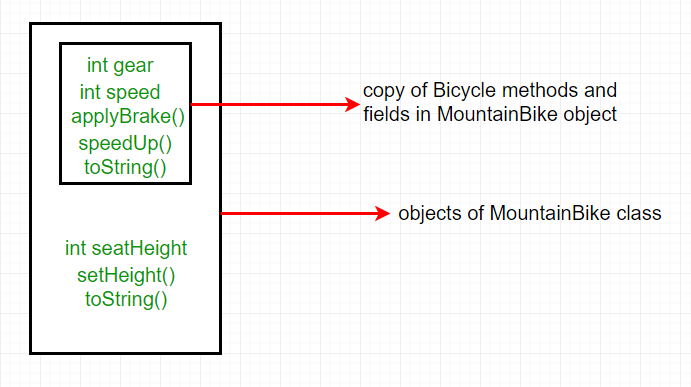In practice, inheritance and polymorphism are used together in java to achieve fast performance and readability of code.

Types of Inheritance in Java

Below are the different types of inheritance which is supported by Java.

1. Single Inheritance : In single inheritance, subclasses inherit the features of one superclass. In image below, the class A serves as a base class for the derived class B.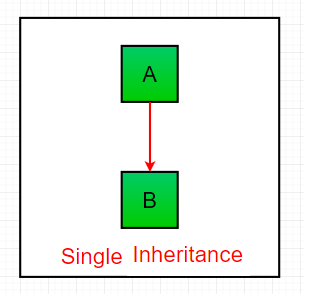`//Java program to illustrate the  ` `// concept of single inheritance ` `import` `java.util.*; ` `import` `java.lang.*; ` `import` `java.io.*; ` ` `  `class` `one ` `{ ` `    ``public` `void` `print_geek() ` `    ``{ ` `        ``System.out.println(``"Geeks"``); ` `    ``} ` `} ` ` `  `class` `two ``extends` `one ` `{ ` `    ``public` `void` `print_for() ` `    ``{ ` `        ``System.out.println(``"for"``); ` `    ``} ` `} ` `// Driver class ` `public` `class` `Main ` `{ ` `    ``public` `static` `void` `main(String[] args) ` `    ``{ ` `        ``two g = ``new` `two(); ` `        ``g.print_geek(); ` `        ``g.print_for(); ` `        ``g.print_geek(); ` `    ``} ` `} `

Output:

```Geeks
for
Geeks
```

2. Multilevel Inheritance : In Multilevel Inheritance, a derived class will be inheriting a base class and as well as the derived class also act as the base class to other class. In below image, the class A serves as a base class for the derived class B, which in turn serves as a base class for the derived class C. In Java, a class cannot directly access the grandparent’s members.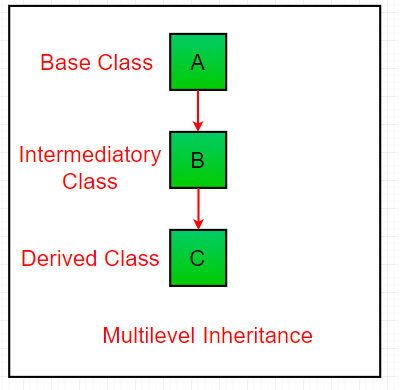`// Java program to illustrate the  ` `// concept of Multilevel inheritance ` `import` `java.util.*; ` `import` `java.lang.*; ` `import` `java.io.*; ` ` `  `class` `one ` `{ ` `    ``public` `void` `print_geek() ` `    ``{ ` `        ``System.out.println(``"Geeks"``); ` `    ``} ` `} ` ` `  `class` `two ``extends` `one ` `{ ` `    ``public` `void` `print_for() ` `    ``{ ` `        ``System.out.println(``"for"``); ` `    ``} ` `} ` ` `  `class` `three ``extends` `two ` `{ ` `    ``public` `void` `print_geek() ` `    ``{ ` `        ``System.out.println(``"Geeks"``); ` `    ``} ` `} ` ` `  `// Drived class ` `public` `class` `Main ` `{ ` `    ``public` `static` `void` `main(String[] args) ` `    ``{ ` `        ``three g = ``new` `three(); ` `        ``g.print_geek(); ` `        ``g.print_for(); ` `        ``g.print_geek(); ` `    ``} ` `} `

Output:

```Geeks
for
Geeks
```
3. Hierarchical Inheritance : In Hierarchical Inheritance, one class serves as a superclass (base class) for more than one sub class.In below image, the class A serves as a base class for the derived class B,C and D.
4.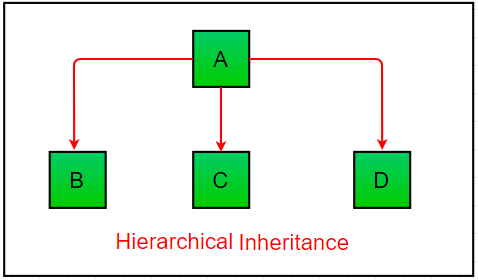`// Java program to illustrate the  ` `// concept of Hierarchical inheritance ` `import` `java.util.*; ` `import` `java.lang.*; ` `import` `java.io.*; ` ` `  `class` `one ` `{ ` `    ``public` `void` `print_geek() ` `    ``{ ` `        ``System.out.println(``"Geeks"``); ` `    ``} ` `} ` ` `  `class` `two ``extends` `one ` `{ ` `    ``public` `void` `print_for() ` `    ``{ ` `        ``System.out.println(``"for"``); ` `    ``} ` `} ` ` `  `class` `three ``extends` `one ` `{ ` `    ``/*............*/` `} ` ` `  `// Drived class ` `public` `class` `Main ` `{ ` `    ``public` `static` `void` `main(String[] args) ` `    ``{ ` `        ``three g = ``new` `three(); ` `        ``g.print_geek(); ` `        ``two t = ``new` `two(); ` `        ``t.print_for(); ` `        ``g.print_geek(); ` `    ``} ` `} `

Output:

```Geeks
for
Geeks
```
5. Multiple Inheritance (Through Interfaces) : In Multiple inheritance ,one class can have more than one superclass and inherit features from all parent classes. Please note that Java does not support multiple inheritance with classes. In java, we can achieve multiple inheritance only through Interfaces. In image below, Class C is derived from interface A and B.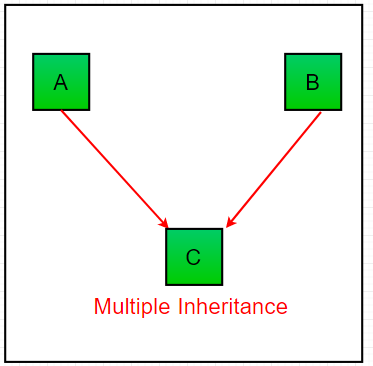`// Java program to illustrate the  ` `// concept of Multiple inheritance ` `import` `java.util.*; ` `import` `java.lang.*; ` `import` `java.io.*; ` `  `  `interface` `one ` `{ ` `    ``public` `void` `print_geek(); ` `} ` `  `  `interface` `two ` `{ ` `    ``public` `void` `print_for(); ` `} ` `  `  `interface` `three ``extends` `one,two ` `{ ` `    ``public` `void` `print_geek(); ` `} ` `class` `child ``implements` `three ` `{ ` `    ``@Override` `    ``public` `void` `print_geek() { ` `        ``System.out.println(``"Geeks"``); ` `    ``} ` `  `  `    ``public` `void` `print_for() ` `    ``{ ` `        ``System.out.println(``"for"``); ` `    ``} ` `} ` ` `  `// Drived class ` `public` `class` `Main ` `{ ` `    ``public` `static` `void` `main(String[] args) ` `    ``{ ` `        ``child c = ``new` `child(); ` `        ``c.print_geek(); ` `        ``c.print_for(); ` `        ``c.print_geek(); ` `    ``} ` `} `

Output:

```Geeks
for
Geeks
```
6. Hybrid Inheritance(Through Interfaces) : It is a mix of two or more of the above types of inheritance. Since java doesn’t support multiple inheritance with classes, the hybrid inheritance is also not possible with classes. In java, we can achieve hybrid inheritance only through Interfaces.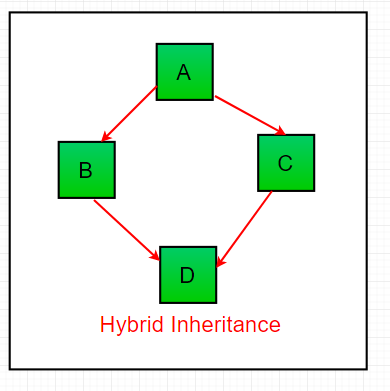Important facts about inheritance in Java

• Default superclass: Except Object class, which has no superclass, every class has one and only one direct superclass (single inheritance). In the absence of any other explicit superclass, every class is implicitly a subclass of Object class.
• Superclass can only be one: A superclass can have any number of subclasses. But a subclass can have only one superclass. This is because Java does not support multiple inheritance with classes. Although with interfaces, multiple inheritance is supported by java.
• Inheriting Constructors: A subclass inherits all the members (fields, methods, and nested classes) from its superclass. Constructors are not members, so they are not inherited by subclasses, but the constructor of the superclass can be invoked from the subclass.
• Private member inheritance: A subclass does not inherit the private members of its parent class. However, if the superclass has public or protected methods(like getters and setters) for accessing its private fields, these can also be used by the subclass.

What all can be done in a Subclass?

In sub-classes we can inherit members as is, replace them, hide them, or supplement them with new members:

• The inherited fields can be used directly, just like any other fields.
• We can declare new fields in the subclass that are not in the superclass.
• The inherited methods can be used directly as they are.
• We can write a new instance method in the subclass that has the same signature as the one in the superclass, thus overriding it (as in example above, toString() method is overridden).
• We can write a new static method in the subclass that has the same signature as the one in the superclass, thus hiding it.
• We can declare new methods in the subclass that are not in the superclass.
• We can write a subclass constructor that invokes the constructor of the superclass, either implicitly or by using the keyword super.My Personal Notes arrow_drop_up

Improved By : sai003, Jitender_1998

Article Tags :
Practice Tags :

62

Please write to us at contribute@geeksforgeeks.org to report any issue with the above content.